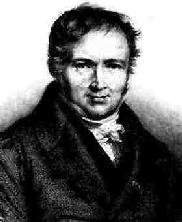# (Week 9 Starting Monday November 20th 2006)

### Hand-in date: one week after your scheduled tutorial session in week 9.

Aims and Objectives: Provide a further opportunity (extending the work carried out on the previous parcatical --- Practical 5) for students to apply their knowledge of loops (pre-test and post-test, and fixed and variable count) using the "for", "while" and/or "do-while" loop constructs taught in weeks 7 and 8.

## 1. REQUIREMENTS

 Given knowledge of the averrage (A) arrival rate of customers at some business (e.g. bank, post office, shop, etc.) the likelyhood of a particular number of customers (X) arriving can be estimated using the Poisson probability function (named after Siméon Denis Poisson): Poisson(X) = (AX * e-A)/X! where X is a number of customer arrivals per minute, A is the average number of arrivals per minute, X! is factorial(X), and e is Euler's number (2.7182818). For example if A = 3 then the probability of one customer entering the premises is: (31 * 2.7182818-3)/1 = 0.149361 Develop a Java software system which takes as input a user-supplied average arrival rate (A), and calculates and displays the Poisson probability associated with a range of values for X (numbers of customers arriving) according to the following: If A (the average number of customers arriving) is greater than five a range of A-5 to A+5 incrementing in steps of 1 (e.g. if A = 7, then X = 2, 3, 4, 5, 6, 7, 8, 9, 10, 11, 12).• Otherwise, becuase we cannot have a negative number of customers arriving, if A is less than or equal to five then a range of 1 to A+5 incrementing in steps of 1 (e.g. if A = 3, then X = 1, 2, 3, 4, 5, 6, 7, 8).

Assume that A is an integer between 1 and 10.

 EXAMPLE 1: Given A=9 (thus A-5 > 0) then the output would be on the following lines: ```Number of | Poisson Customers | Probability (X) | ----------+------------ 4 | 0.033737 5 | 0.060727 6 | 0.091091 7 | 0.117117 8 | 0.131756 9 | 0.131756 10 | 0.118581 11 | 0.097021 12 | 0.072765 13 | 0.050376 14 | 0.032384 ``` EXAMPLE 2: Given A=2 (thus A-5 <= 0) the output will be of the form: ```Number of | Poisson Customers | Probability (X) | ----------+------------ 1 | 0.270671 2 | 0.270671 3 | 0.180447 4 | 0.090224 5 | 0.036089 6 | 0.012030 7 | 0.003437 ```
 Note 1: You should build at least two classes, one that characterises the "Poisson probability" problem in general and an application class. Note 2: To calculate a X! (factorial X) make use of the Factorial class presented in lectures (week 7). To use this you will have to copy (cut and past!) the class into the same directory as your other classes used to address this practical. Once compiled you can create intances of the Factorial class which may be of use in the solution to the current problem. (Assume that the Factorial has been properly designed and tested and thuys will not rerquire any further design or testing,) Note 3: Euler's constant can be found in the Math class where it is defined as a class field and can thus be accessed by linking it to the name of the class, Math.E.Siméon Denis Poisson (1781 - 1840) 1

## 2. REPORT

Your solution should comprise the following:

1. A report in the form of a single Microsoft Word file.
2. The Java source files of your implementation.

Your report should comprise the following sections:

1. Requirements: Summary of the above.
2. Analysis: Your analysis of the problem comprising: (i) a Class Diagram outlining the proposed class structure/hierarchy, and (ii) a set of summary tables describing the fields, constructors and methods for each class you intend to create.
3. Design: Detailed designs for the methods you intend to include, described using Nassi-Shneiderman charts, and a high-level Activity Diagram describing the "paths" through your code.
4. Implementation: A computer print out of your implementation.
5. Testing: A set of appropriate test cases together with the output from your program as a result of running these test cases (refer to your Activity Diagram when drawing up your path and loop test cases).

All supporting documentation should be prepared as a single Microsoft Word file.

## 3. SUBMISSION

Once completed you should "up load" your Java source files (extension .java) and your word document to the CS department's electronic "practical assignment submission" system.

## 4. MARK SCHEME

Marks will be awarded for:

1. Analysis and design (30%)
2. Implementation (30%)
3. Testing (30%)
4. Write Up (10%)

With the total number of available marks distributed as indicated.

Remember:

1. Guidance notes for the execution of COMP101 practicals.
2. General guidance notes on COMP101 practicals and course work with respect to: the presentation of work, the COMP101 marking scheme and University's late submission policy.
3. It is better to hand in an incompleted piece of work rather than nothing at all as this will result in some marks being awarded, while the latter option is guaranteed to result in a mark of 0!

## 4. REFERENCES

1. W. W. Rouse Ball (1908), A Short Account of the History of Mathematics: Siméon Denis Poisson (1781 - 1840), Hosted by Trinity College, Dublin ( http://www.maths.tcd.ie/pub/HistMath/.

Created and maintained by Frans Coenen. Last updated 30 October 2006